International
Tables for
Crystallography
Volume C
Mathematical, physical and chemical tables
Edited by E. Prince

International Tables for Crystallography (2006). Vol. C, ch. 6.4, p. 610

## Section 6.4.4. Radiation flow

T. M. Sabinea

aANSTO, Private Mail Bag 1, Menai, NSW 2234, Australia

### 6.4.4. Radiation flow

| top | pdf |

The radiation flow is governed by the Hamilton–Darwin (H–D) equations (Darwin, 1922; Hamilton, 1957). These equations are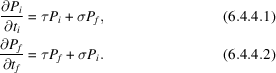Here,is the radiation current density (m−2 s−1) in the incident (i = initial) beam,is the current density in the diffracted (f = final) beam. The distancesand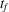are measured along the incident and diffracted beams, respectively. The coupling constant σ is the cross section per unit volume for scattering into a single Bragg reflection, while τ, which is always negative, is the cross section per unit volume for removal of radiation from the beams by any process. In what follows, it will be assumed that absorption is the only significant process, and τ is given by τ = −(σ + μ), where μ is the linear absorption coefficient (absorption cross section per unit volume). This assumption may not be true for neutron diffraction, in which incoherent scattering may have a significant role in removing radiation. In those cases, τ should include the incoherent scattering cross section per unit volume.

The H–D equations have analytical solutions in the Laue case (2θ = 0) and the Bragg case (2θ = π). The solutions at the exit surface are, respectively,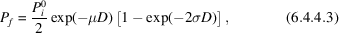and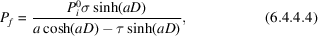with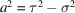. The path length of the diffracted beam through the crystal is D. The current density at the entrance surface is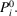To find formulae for the integrated intensity, it is necessary to express σ in terms of crystallographic quantities.

### References

Darwin, C. G. (1922). The reflexion of X-rays from imperfect crystals. Philos. Mag. 43, 800–829.
Hamilton, W. C. (1957). The effect of crystal shape and setting on secondary extinction. Acta Cryst. 10, 629–634.